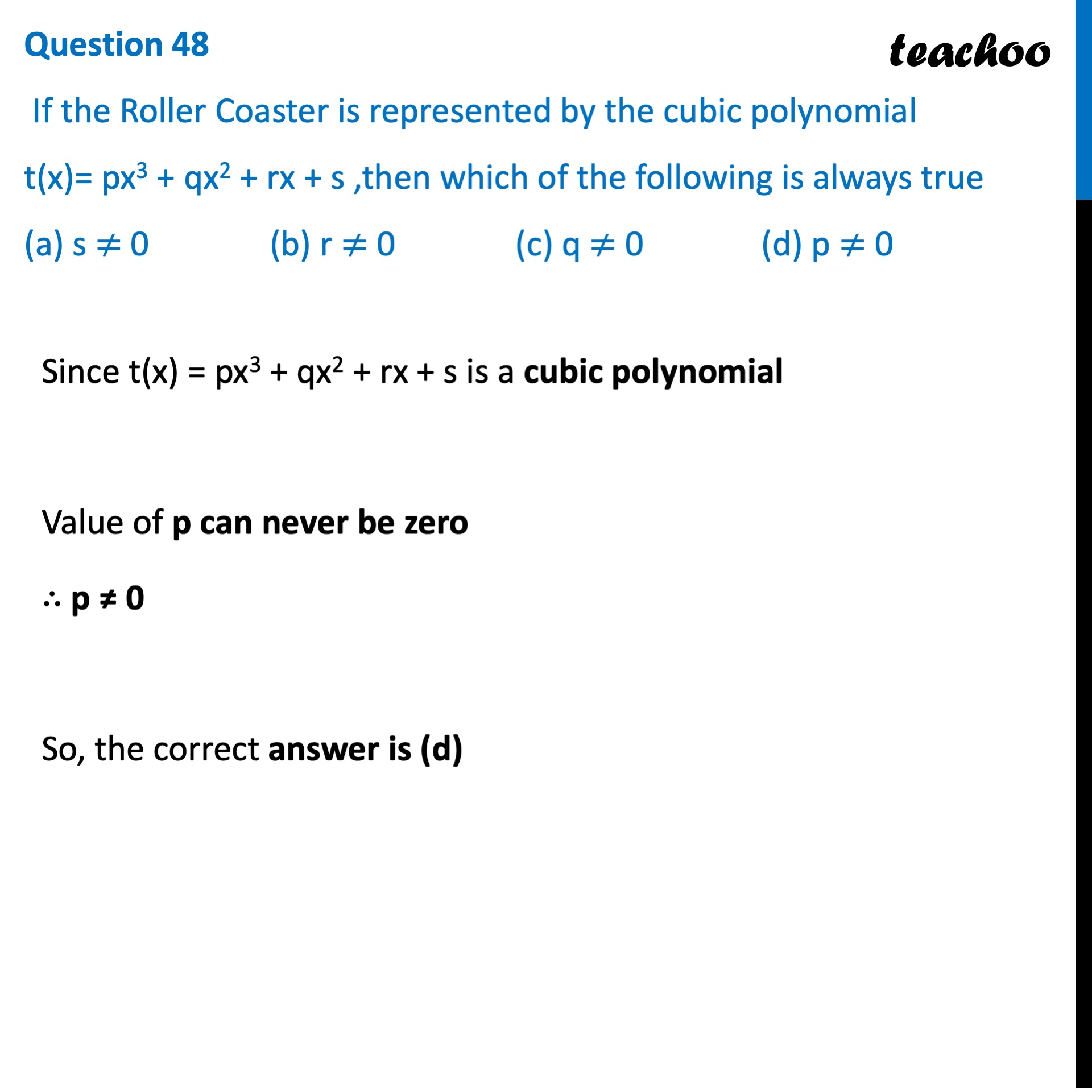## (a) s ≠ 0   (b) r ≠ 0   (c) q ≠ 0   (d) p ≠ 01. Class 10
2. Solutions of Sample Papers for Class 10 Boards
3. CBSE Class 10 Sample Paper for 2022 Boards - Maths Basic [MCQ]

Transcript

Question 48 If the Roller Coaster is represented by the cubic polynomial t(x)= px3 + qx2 + rx + s ,then which of the following is always true (a) s ≠ 0 (b) r ≠ 0 (c) q ≠ 0 (d) p ≠ 0 Since t(x) = px3 + qx2 + rx + s is a cubic polynomial Value of p can never be zero ∴ p ≠ 0 So, the correct answer is (d)